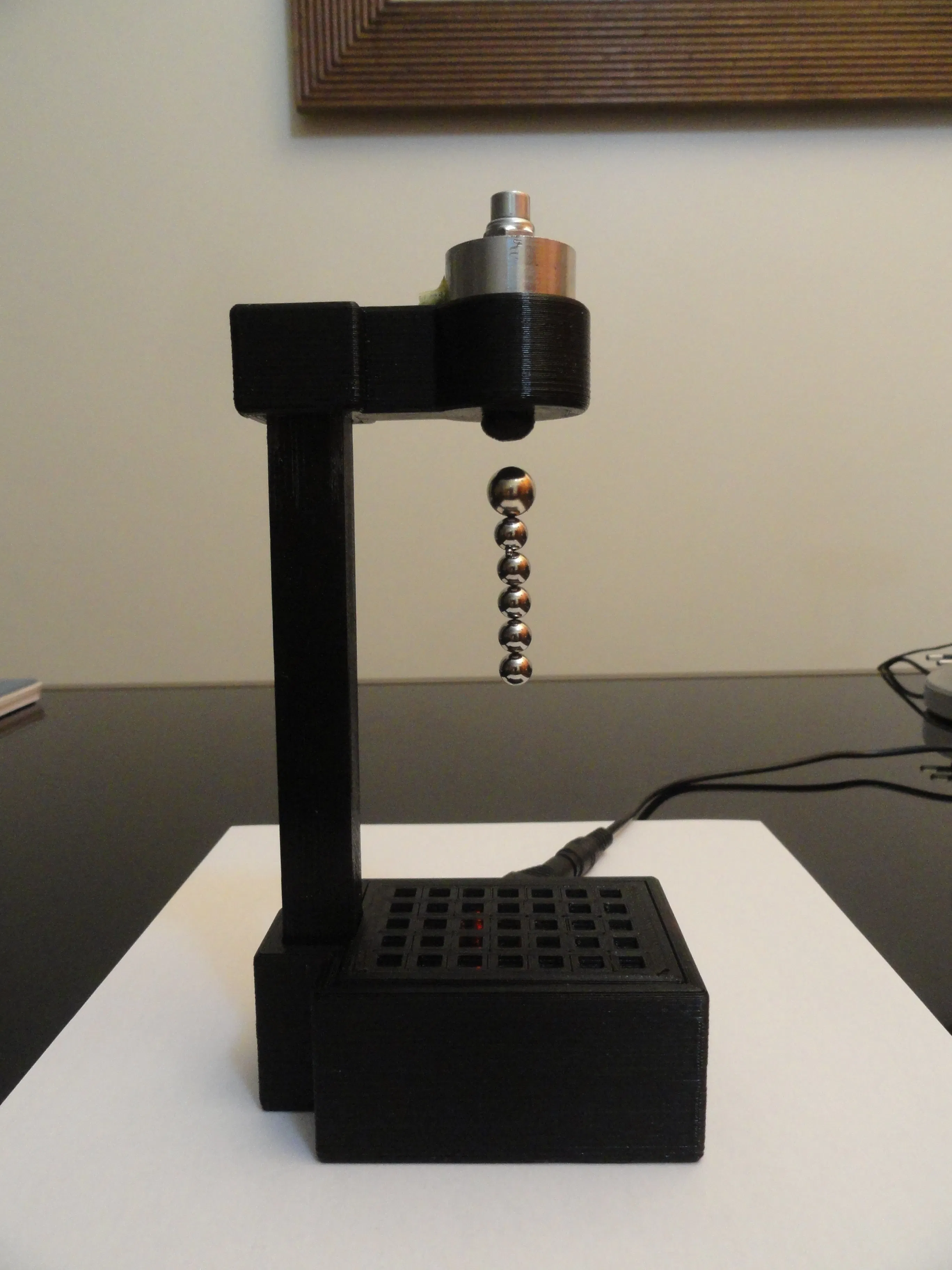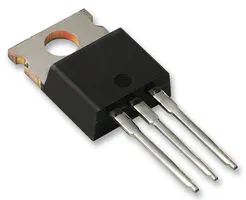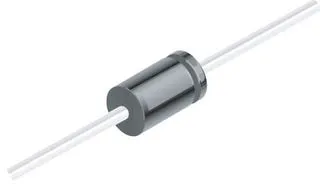# Magnet Levitation with Arduino

Magnet levitation toy using Arduino. Cheap and easy to make!

IntermediateFull instructions provided147,160## Things used in this project

### Hardware componentsArduino Nano R3 You can use any arduino, but the shield is made for the Nano.
×1
 Electromagnet 12v 25mm diameter.
×1
 Linear Hall Effect Sensor 49E Do not use hall effect switch.
×1Darlington High Power Transistor TIP 120
×1Resistor 1k ohm
×11N4007 – High Voltage, High Current Rated Diode
×1
 Power supply 12V 1A
×1×1SparkFun Pushbutton switch 12mm
×2Jumper wires (generic)
×1

### Software apps and online servicesArduino IDE

## Schematics

### Board Schematic

Fritzing IDE## Code

### Levitator.ino

Arduino
Code for Magnet Levitation toy with Arduino.
Easy(No PID)
```//=========================================================|
//     Ekobots Innovation Ltda - www.ekobots.com.br        |
//        Juan Sirgado y Antico - www.jsya.com.br          |
//---------------------------------------------------------|
//        Program Magnetic Levitator - 2016/02/06          |
//=========================================================|
int anaPin = 1;   // Arduino Analogic Pin 1;
int digPin = 5;   // Arduino Digital  Pin 5;
int subPin = 7;   // Arduino Digital  Pin 7;
int addPin = 8;   // Arduino Digital  Pin 8;
//
int     anaVal = 0;   // Analogic Read(Hall Sensor) Value;
boolean digVal = 0;   // Digital Write(Electromagnet) Value;
//
int levVal = 228;   // Levitation Poit Value;
int dlyVal = 250;   // Delay Value Micro Seconds;
//---------------------------------------------------------|
void setup()
{
// Levitator initialization Begin;
Serial.begin(57600);
Serial.println("Levitator by JSyA");
Serial.println("Starting...");
// Digital Pins Work Mode Setup;
pinMode(digPin, OUTPUT);
pinMode(subPin, INPUT_PULLUP);
// Levitator initialization End;
Serial.println("Started.");
}
//---------------------------------------------------------|
void loop()
{
// Hall Sensor Read (Magnetic Field Intensity);
// Increase The Value Of Levitation Point;
{
levVal++;
value_log();
delay(250);
}
// Decrease The Value Of Levitation Point;
{
levVal--;
value_log();
delay(250);
}
// Check the Levitation Point;
if (anaVal < levVal)
{
digVal = LOW;
}
else // if (anaVal > levVal)
{
digVal = HIGH;
}
//
// Turn ON/OFF The Electromagnet;
// With Base on Sensor Value and the Levitation Point;
digitalWrite(digPin, digVal);
delayMicroseconds(dlyVal);
}
//---------------------------------------------------------|
void value_log()
// Analogic/Digital/Levitation Values Print;
{
// Show the Hall Sensor Value;
Serial.print("anaVal=[");
Serial.print(anaVal);
Serial.print("]-");
// Show the Electromagnet state On=1/Off=0;
Serial.print("digVal=[");
Serial.print(digVal);
Serial.print("]-");
// Show the Levitation Point Value;
Serial.print("levVal=[");
Serial.print(levVal);
Serial.println("];");
}
//=========================================================|
```

### Levitator_PID.ino

Arduino
Code for Magnet Levitation toy with Arduino.
Hard(PID version)
```//=========================================================|
//     Ekobots Innovation Ltda - www.ekobots.com.br        |
//        Juan Sirgado y Antico - www.jsya.com.br          |
//---------------------------------------------------------|
//      Program Magnetic Levitator PID - 2016/10/06        |
//=========================================================|
int anaPin = 1;   // Arduino Analogic Pin 1
int digPin = 5;   // Arduino Digital  Pin 5
int subPin = 7;   // Arduino Digital  Pin 7
int addPin = 8;   // Arduino Digital  Pin 8
//
int anaVal = 0;   // Analogic Valie
int digVal = 0;   // Digital Value
//
int levVal = 262; // Levitation Value
int dlyVal = 10;  // Delay Value
//
float timVal = 0; // Time Value
//---------------------------------------------------------|
// PID Values
float setpoint = 0;
float measured_value = 0;
float output = 0;
float integral = 0;
float derivative = 0;
float error = 0;
float previous_error = 0;
float dt = 0.1;
float Kp = 1.0;
float Ki = 0.1;
float Kd = 0.01;
//---------------------------------------------------------|
void setup()
{
// Levitator initialization Begin;
Serial.begin(57600);
Serial.println("Levitator by JSyA");
Serial.println("Starting...");
// Digital Pins Work Mode Setup;
pinMode(digPin, OUTPUT);
pinMode(subPin, INPUT_PULLUP);
//
timVal = millis();
setpoint = levVal;
// Levitator initialization End;
Serial.println("Started.");
}
//---------------------------------------------------------|
void loop() // PID
{
// Hall Sensor Read (Magnetic Field Intensity);
// PID calculations
measured_value = anaVal;
error = setpoint - measured_value;
integral = integral + error * dt;
derivative = (error - previous_error) / dt;
output = (-Kp * error) + (-Ki * integral) + (-Kd * derivative);
previous_error = error;
// Final value setup
digVal += output;
// Check the value for levitation point;
if (digVal < 0) digVal=0;
if (digVal > 255) digVal=255;
// Increase/Decrease the value for Electromagnet;
// With Base on Sensor Value and the Levitation Point;
analogWrite(digPin, digVal);
// Show log values for debug;
//   if((millis()-timVal) > 500)
//   {
//      value_log();
//      timVal = millis();
//   }
// Increase The Value Of Levitation Point;
{
setpoint++;
value_log();
delay(250);
}
{
setpoint--;
value_log();
delay(250);
}
// Time between electromagnet state changes;
delayMicroseconds(dlyVal);
}
//---------------------------------------------------------|
void value_log()
// Analogic/Digital/Levitation Values Print;
{
// Show the Hall Sensor Value;
Serial.print("anaVal=[");
Serial.print(anaVal);
Serial.print("]-");
// Show the Electromagnet state On=1/Off=0;
Serial.print("digVal=[");
Serial.print(digVal);
Serial.print("]-");
// Show the Levitation Point Value;
Serial.print("setpoint=[");
Serial.print(setpoint);
Serial.println("];");
}
//=========================================================|
```